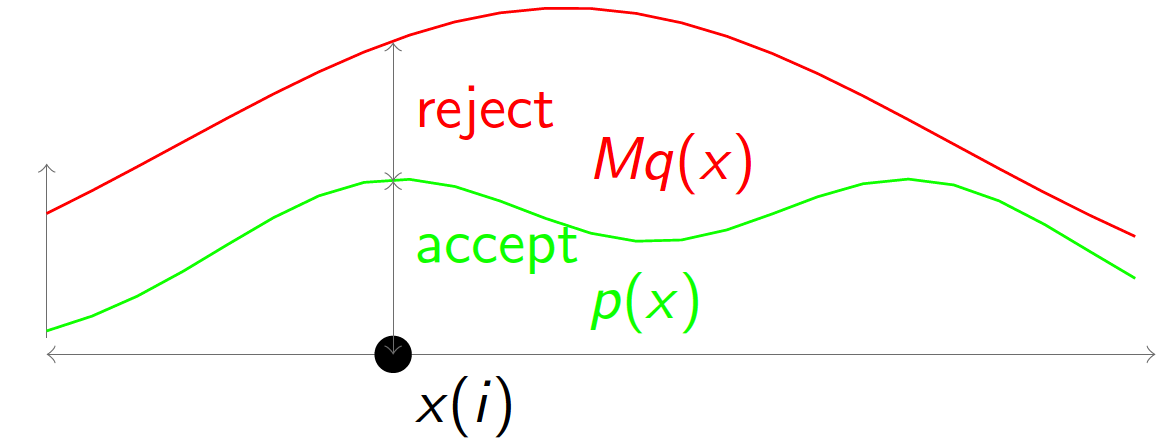# 470. Implement Rand10() Using Rand7() （拒绝采样Reject Sampling）

### 2. 思路

（1）通过(Rand N - 1) % 10 + 1的方法，可以求出Rand10，当N是10的倍数的时候。
（2）用( Rand7 - 1 ) * 7 + Rand7可以随机生成1-49，记作Rand49。
（3）如果可以通过Rand49计算出Rand40，即随机生成1-40，就可以通过Rand40 % 10来取得Rand10。
（4）如何通过Rand49计算出Rand40呢？可以通过拒绝采样的方法来计算：用Rand49生成一个数，如果它位于41-49，则丢弃，继续生成，当生成一个1-40的数时返回。这样子做可以近似看成随机生成1-40之间的数。
（5）到这里就可以把问题解决了，下面是一些扩展知识。
（6）深入思考一下，为什么这样子做是有用的呢？下面详细阐述一下拒绝采样回到本题的一个思路历程。

（1）将q(x)和一个大于1的常数M相乘，使得M * q(x)可以把p(x)罩住，如下图所示（图源见参考文献）。

（2）从q(x)中获得一个采样点x(i)，对于x(i)计算一个接受概率 $\alpha = \frac{p(x_i)}{Mq(x_i)}$，从Uniform(0,1)随机生成一个值$\mu$，如果$\alpha \ge \mu$，则接受x(i)作为一个来自p(x)的采样点。否则继续采样。Rand7以随机生成1-7，那么用( Rand7 - 1 ) * 7 + Rand7就可以随机生成1-49，记作Rand49()。

rand7调用次数的期望值：2.45，计算如下

$\begin{split}E &= 2 \cdot \frac{40}{49} + 4 \cdot \frac{9}{49} \cdot \frac{40}{49} + 6 \cdot (\frac{9}{49})^2 \cdot \frac{40}{49} + ... + 2k \cdot (\frac{9}{49})^{k-1} \cdot \frac{40}{49} \\ &= \frac{80}{49} ( 1 + 2 \cdot \frac{9}{49} + 3 \cdot (\frac{9}{49})^2 + ... + \ k \cdot (\frac{9}{49})^{k-1} ), \ k \to \infty \\ \frac{49}{80} E &= 1 + 2 \cdot \frac{9}{49} + 3 \cdot (\frac{9}{49})^2 + ... + \ k \cdot (\frac{9}{49})^{k-1} \\ ( \frac{49}{80} \cdot \frac{9}{49} ) \ E &= \frac{9}{49} + 2 \cdot (\frac{9}{49})^2 + 3 \cdot (\frac{9}{49})^3+ ... + \ (k-1) \cdot (\frac{9}{49})^{k-1} + k \cdot (\frac{9}{49})^{k} \\ ( \frac{49}{80} - \frac{49}{80} \cdot \frac{9}{49} ) \ E &= 1 + \frac{9}{49} + (\frac{9}{40})^2 + (\frac{9}{49})^3+ ... + (\frac{9}{49})^{k-1} - k \cdot (\frac{9}{49})^{k} \\ \frac{1}{2} \ E &= \frac{1 - (\frac{9}{49})^k}{1 - \frac{9}{49}} - k \cdot (\frac{9}{49})^{k}\end{split}$

（方法二）利用被拒绝的数

rand7调用次数的期望值：2.19

$\begin{split} E =\,& 2 \cdot \frac{40}{49} +3 \cdot \frac{9}{49} \cdot \frac{60}{63} + 4 \cdot \frac{9}{49} \cdot \frac{3}{63} \cdot \frac{20}{21} + \\ &\left(\frac{9}{49} \cdot \frac{3}{63} \cdot \frac{1}{21}\right) \times \left( 6 \cdot \frac{40}{49} + 7 \cdot \frac{9}{49} \cdot \frac{60}{63} + 8 \cdot \frac{9}{49} \cdot \frac{3}{63} \cdot \frac{20}{21} \right) + \\ &\left(\frac{9}{49} \cdot \frac{3}{63} \cdot \frac{1}{21}\right)^2 \times \left( 10 \cdot \frac{40}{49} + 11 \cdot \frac{9}{49} \cdot \frac{60}{63} + 12 \cdot \frac{9}{49} \cdot \frac{3}{63} \cdot \frac{20}{21} \right) + \\ &\ldots \\ &\left(\frac{9}{49} \cdot \frac{3}{63} \cdot \frac{1}{21}\right)^k \times \left( (2+4k) \cdot \frac{40}{49} + (3+4k) \cdot \frac{9}{49} \cdot \frac{60}{63} + (4+4k) \cdot \frac{9}{49} \cdot \frac{3}{63} \cdot \frac{20}{21} \right), k \to \infty \\ =\,& 2.19 \end{split}$

### 3. 代码

# The rand7() API is already defined for you.
# def rand7():
# @return a random integer in the range 1 to 7

class Solution(object):
def rand10(self):
"""
:rtype: int
"""
while True:
val = (rand7() - 1) * 7 + rand7()
if(val <= 40):
break
return (val-1) % 10 + 1

class Solution(object):
def rand10(self):
"""
:rtype: int
"""
while True:
val = (rand7() - 1) * 7 + rand7()
if val <= 40:
return (val-1) % 10 + 1
val -= 40
val = (val - 1) * 7 + rand7()
if val <= 60:
return (val-1) % 10 + 1
val -= 60
val = (val - 1) * 7 + rand7()
if val <= 20:
return (val-1) % 10 + 1


### 5. 参考资料

posted @ 2018-10-26 16:42  PilgrimHui  阅读(464)  评论(0编辑  收藏